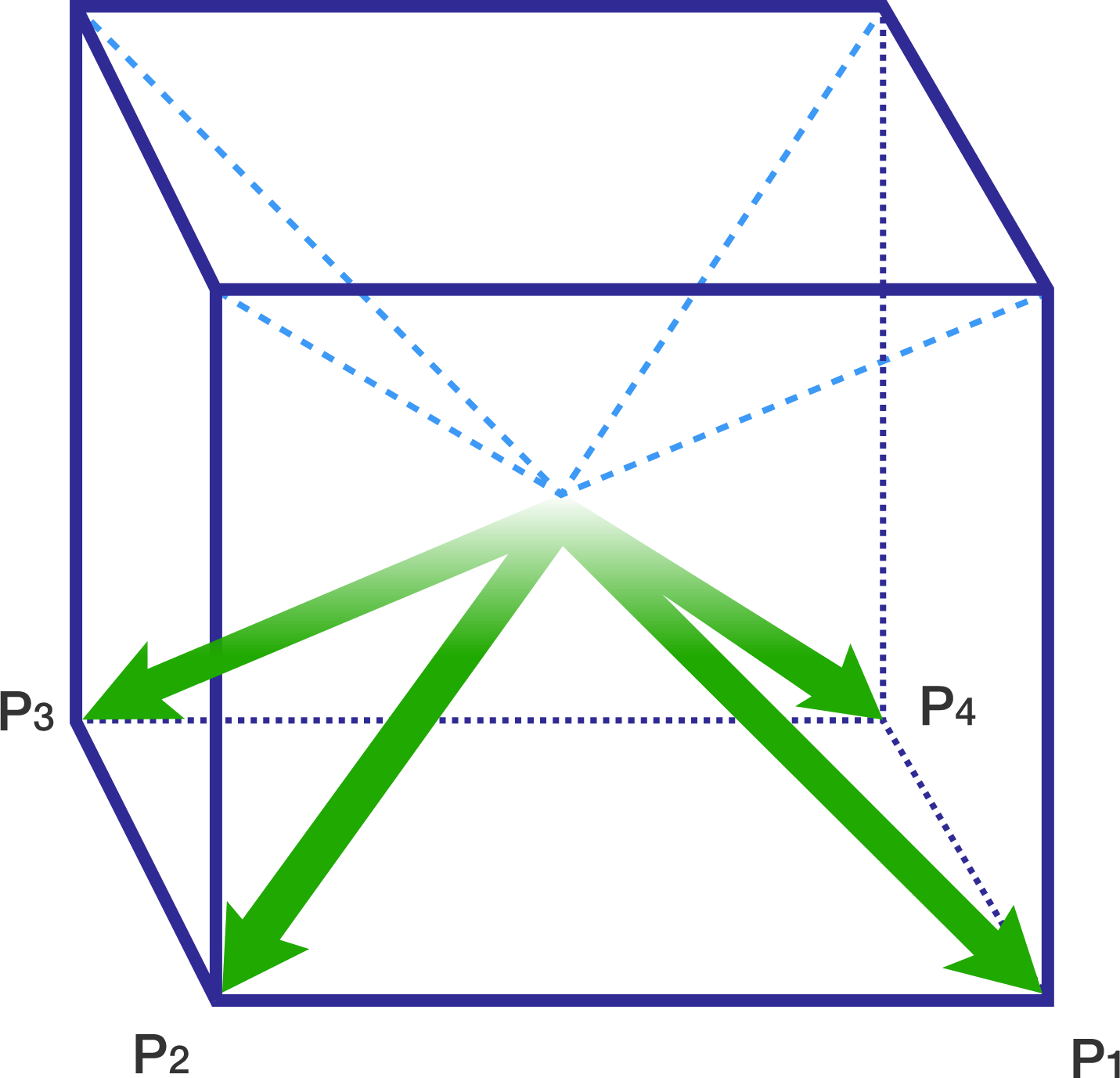# Diagonals of a Cube

Geometry Level 2If a line makes angles $\alpha$ , $\beta$ , $\gamma$ and $\delta$ with the four body diagonals of a cube and the value of
$\cos^2(\alpha) + \cos^2(\beta) + \cos^2(\gamma) + \cos^2(\delta)$ can be expressed as $\frac{p}{q}$, where $p$ and $q$ are coprime integers, find the value of $p + q$.

Clarification: Body diagonals of a cube are the diagonals which do not lie along any face of the cube.

×

Problem Loading...

Note Loading...

Set Loading...